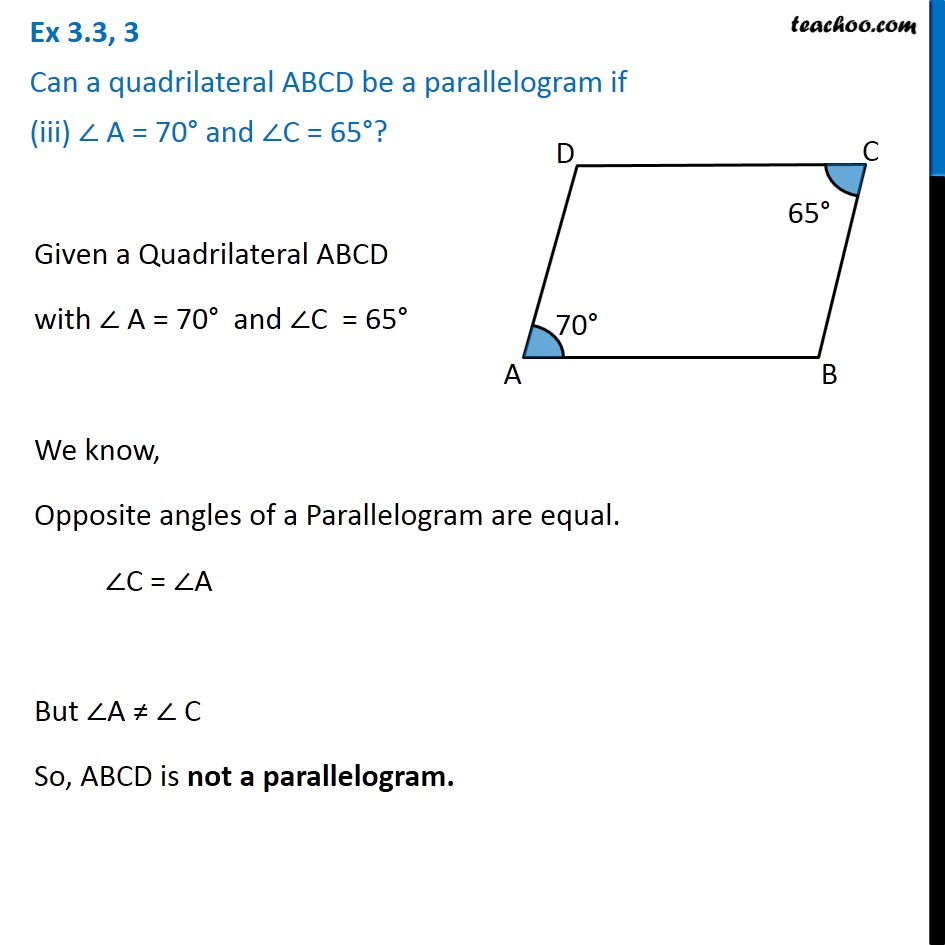1. Chapter 3 Class 8 Understanding Quadrilaterals
2. Serial order wise
3. Ex 3.3

Transcript

Ex 3.3, 3 Can a quadrilateral ABCD be a parallelogram if (iii) ∠ A = 70° and ∠C = 65°?Given a Quadrilateral ABCD with ∠ A = 70° and ∠C = 65° We know, Opposite angles of a Parallelogram are equal. ∠C = ∠A But ∠A ≠ ∠ C So, ABCD is not a parallelogram.